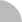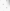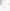##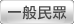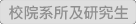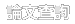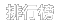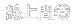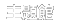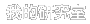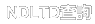(3.238.173.209) 您好！臺灣時間：2021/05/16 21:32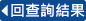### 詳目顯示:::

: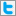Twitter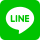•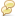被引用:0
•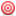點閱:21
•評分: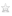•下載:1
•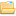書目收藏:0
 這篇碩士論文要是研究仿射平面曲線的交點數。事實上，我們將張海潮教授和王立中教授在[CW]的論述中，歸納並得出以下我們的主要定理:「如果曲線F(1,y,z)在無窮遠處只有一個place，則我們可以建構出與曲線F(1,y,z)相交的曲線G_j，使得它們在所有曲線上達到最小的正交點數。」這是應用到Bezout定理，以及在[Moh1, Moh2, Moh3, Moh4]介紹的近似根概念。此外，我們可以將Embedding Line Theorem作為一個應用並加以證明。(請參閱第八章)
 In this thesis, we study the intersection number of affine plane curves.Actually, we generalize the argument of Chang and Wang in [CW] to obtain our main theorem as follows:“if the curve \$F(1,y,z)\$ has only one place at infinity, then we would construct a curve G_j which intersects curve F(1,y,z) attaining the positive minimal intersection number among all curves."This is an application of Bezout's Theorem and the approximate roots introduced by [Moh1, Moh2, Moh3, Moh4].Besides, we can reprove the Embedding Line Theorem as an application (see section 8).
 1 Introduction........................................12 Basic Knowledge of Commutative Algebra..............22.1 Ideals and Modules.................................22.2 Discrete Valuation Ring............................33 Fundamental Knowledge of Algebraic Curves...........43.1 Affine Algebraic Sets and Affine Varieties.........43.2 The Intersection Properties of Affine Plane Curves.53.3 Projective Varieties...............................63.4 Bezout’s Theorem...................................84 Parametrizations and Places........................144.1 Parametrizations of Curves........................144.2 Places of Curves..................................154.3 Discussion and Example............................155 Zariski’s Works....................................186 The Approximate Root of Polynomials................206.1 Definitions.......................................206.2 Applications of Polynomials.......................207 Main Theorem.......................................248 Embedding Line Theorem.............................279 Appendix...........................................29Reference..............................................30
 Newton-Puiseux expansion and generalized Tschirnhausen transformation. I, II;Embeddings of the line in the plane;Lectures on expansion techniques in algebraic geometry;On equisingularity, analytical irreducibility and embedding line theorem;An Intersection Theoretical Proof of the Embedding Line Theorem;Algebraic Curves : An introduction to Algebraic Geometry;Algebraic Geometry;On Abhyankar-Moh's epimorphism theorem:Embeddings of the plane;Commutative Ring Theory;Curves on Rational and Irrational Surfaces;On the concept of approximate roots for algebra;On characteristic pairs of algebroid plane curves for characteristic p;On two fundamental theorems for the concept of approximate roots;Algebra 3rd ed.;An Algebraic Introduction to Complex Projective Geometry : Commutative Algebra;Algebraic Curves;Le problème des modules pour les branches planes;Commutative Algebra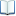電子全文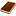國圖紙本論文
 連結至畢業學校之論文網頁點我開啟連結註: 此連結為研究生畢業學校所提供，不一定有電子全文可供下載，若連結有誤，請點選上方之〝勘誤回報〞功能，我們會盡快修正，謝謝！推文當script無法執行時可按︰推文 網路書籤當script無法執行時可按︰網路書籤 推薦當script無法執行時可按︰推薦 評分當script無法執行時可按︰評分 引用網址當script無法執行時可按︰引用網址 轉寄當script無法執行時可按︰轉寄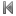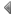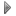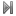top
 無相關論文

 無相關期刊

 無相關點閱論文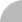簡易查詢 | 進階查詢 | 熱門排行 | 我的研究室